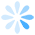### 字符串搜索：ZBox算法

精确字符串匹配的问题定义： 在文本串 T 中搜索与查询串 P 精确匹配的所有子串。

精确字符串匹配问题是一个非常古老且很经典的问题，是字符串搜索技术的基础。最原始也最简单的方法是使用蛮力算法（Brute Force algorithm），蛮力算法在 T 中从头到尾，与 P 比一遍，把与 P 精确匹配的子串输出。这种做法的时间复杂度是 O(n*m)，假设T的长度是 m , P 的长度是 n 。因为出现了很大数量的重复比较，所以效率比较低。因此需要出现了非常多字符串搜索算法，Zbox就是其中一种。

Zbox 算法中没有重复比较，时间复杂度做到了线性 O(n+m)。当然，这不是最好的，在其他的字符串搜索算法中可以做到时间复杂度低于线性。

Zbox 的意思：一个字符串 S ，它的子串 S[i..n], 用 Z[i] 表示 S[i..n] 与 S 精确匹配的最长前缀的长度。如：abcdabce ,Z = 3。
如图 1：那么如何把 S 中所有的 Z[i] 找出来呢？并且让它的时间是线性的。
如图 2：Z = 31，求 Z = ?
可以看出，因为 Z = 31, 所以 S[100..130] 与 S[1..31] 是相同的，显然 S[22..31] 与 S[121..130] 也是相同的。那么，是不是可以通过 Z 来求 Z 呢？
答案是，当 Z < 10 的时候，Z = Z; 而当 Z >= 10的时候，Z <= Z, Z真正的值，还需要通过比较 S[131..n] 这些后面的字符才能算出。
由上面的例子得出 Zbox 算法：
设 k 为 S 的任意一个位置，循环从 k=2 到 k=n-1。
设 r 是 当前 Zbox 覆盖的最靠右的位置，l 是 当前 r 所属的 Zbox的左起点。
1. 若 k > r, 则 k 未落在当前覆盖最远的 Zbox 中，所以不能用现成的 Z[i] 值，只好老老实实地比较 S[1..n] 和 S[k..n]，直到不能匹配的位置 q ，则 Z[k] = q-k ，l = k，r = q-1。
2. 若 k <= r, 则 k 落在了当前覆盖最远的 Zbox 中， 所以可以利用上之前已经计算好的 Z[i] 值。但是还要分两种情况。设 g=r-k+1。如图2。
a. 如果 Z[k'] < g ，则 Z[k]=Z[k'];
b. 如果 Z[k'] >= g，则需要从第 r+1 个字符开始检验，直到不能匹配的字符q，则 Z[k] = q-k ，l = k ，r = q-1 。

利用上述 Zbox 算法，就可以在文本 T 中发现所有与 p 精确匹配的子串了。方法是，把 P 排列在 T 前，并在其分界处插入字符集以外的字符，如 \$ 。
如图 3：这样，找到所有 Z[i]，当 i > |P|+1 ，且 Z[i] = |P|时，位置i就是一个与 P 精确匹配的位置，如此继续下去直到找到所有位置。
时间复杂度分析：因为 T 和 P 的字符至多被比较了一次，所以时间复杂度是 O(m+n)，|P| = m，|O| = n。
空间复杂度分析：因为 \$ 不会出现在 T 和 P 中，所以 Z[i] <= |P|， 这样，存储在 T 中的 i 的 Z[i] 值是没有用的。因此得出空间复杂度是 O(m)。

Friday, March 01, 2013 | 其他技术

#### 文章评论

• ##### #re: 字符串搜索：ZBox算法
如果一个字符串为：AAAAAAAAAAAAA
Z(5)的多少？5还是10？？
• ##### #re: 字符串搜索：ZBox算法
如果一个字符串为：AAAAAAAAAAAAA
Z(5)的多少？5还是10？？
我的理解是10.